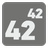Index Power
Elementary
English CS DE EL ES FA FR IT JA PL PT-BR RU UK

You are given an array with positive numbers and a number N. You should find the N-th power of the element in the array with the index N. If N is outside of the array, then return -1. Don't forget that the first element has the index 0.

Let's look at a few examples:
- array = [1, 2, 3, 4] and N = 2, then the result is 32 == 9;
- array = [1, 2, 3] and N = 3, but N is outside of the array, so the result is -1.

Input: Two arguments. An array as a list of integers and a number as a integer.

Output: The result as an integer.

Example:

```index_power([1, 2, 3, 4], 2) == 9
index_power([1, 3, 10, 100], 3) == 1000000
index_power([0, 1], 0) == 1
index_power([1, 2], 3) == -1
```

How it is used: This mission teaches you how to use basic arrays and indexes when combined with simple mathematics.

Precondition: 0 < len(array) ≤ 10
0 ≤ N
all(0 ≤ x ≤ 100 for x in array)